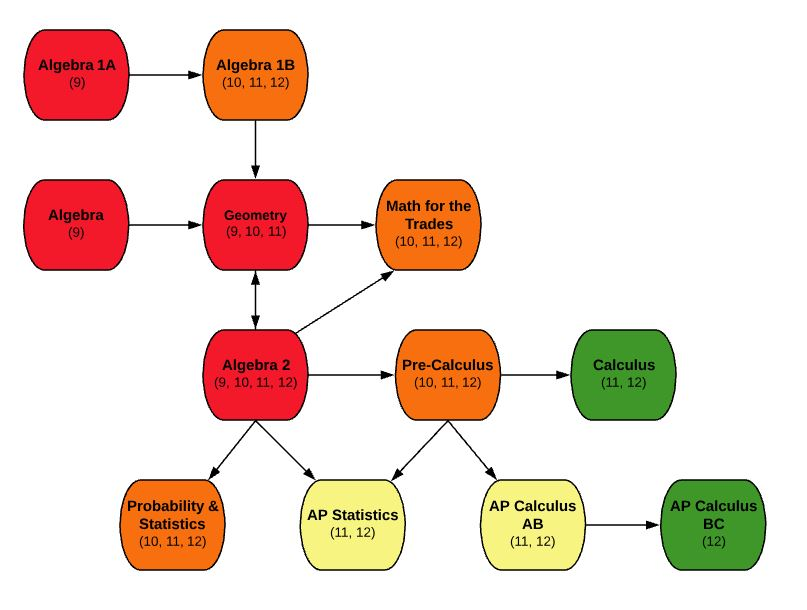EDIT MAIN

# MA414 Pre-Calculus10, 11, 12

Mathematics

Standard

1

This is a year long course that requires selection of both semesters: S1 and S2. This course will explore functions and their behavior and to prepare students for advanced study in mathematics. This course will explore Linear, Polynomial, Rational, Exponential, Logarithmic and Trigonometric Functions. The graphs of these functions will be studied and graphing calculators will be used to further develop an understanding of these functions and graphs. Systems of equations and inequalities will also be studied. A TI-83 or TI-84 graphing calculator is required for this course.

Outcomes:

• Graph piecewise functions, rational functions, linear and polynomial inequalities
• Solve systems of equations by using the graphing calculator
• Use linear programming procedures to solve real-world applications
• Determine the continuity, end behavior, critical points and extrema of relations using the graphing calculator.
• Locate zeros of polynomial functions
• Use the unit circle to determine the value of trigonometric functions
• Find side or angle measure by using the law of sines and the law of cosines
• Graph and identify important characteristics of trigonometric function
• Verify trigonometric identities including sum, difference, double-angle, and half-angle identities
• Solve trigonometric equations
• Solve exponential and logarithmic equations
• Describe characteristics of the arithmetic, geometric, and infinite series
• Determine whether series are convergent or divergent
• Count sample spaces by using permutations and combinations
• Use matrices to solve systems of equations
• Compute probabilities including compound events and conditional probabilities

Minimum grade of C- in Algebra 2

If you take this course, you must also take MA414-S2 – Pre-Calculus S2

A TI-83 or TI-84 graphing calculator or comparable is required for this course.